### No Spooky Actions at a Distance 2005 Brilliant Light Power, Inc.

The Aspect experiment is often invoked as the proof of the quantum-mechanical nature of reality [1-9] wherein nothing in the universe (e.g. photons, electrons, neutrons, atoms), nor the universe itself, has a definite form until it is measured. An even more disturbing prediction of quantum mechanics is that a measurement of a quantum entity can instantaneously influence another light years away. Einstein, Podolsky, and Rosen (EPR) in a classic paper  argued that such a consequence proved that Standard Quantum Mechanics (SQM) was not a complete theory, but it could be corrected by a reinterpretation of SQM predictions as outcomes of existence of “hidden variables,” the specification of which would predetermine the result of measuring any observable of the physical system. Bell showed that no local hidden-variable theory could reproduce all of the statistical predictions of SQM. Thus, a paradox arises from Einstein’s conviction that SQM predictions concerning spatially separated systems are incompatible with the condition for locality unless hidden variables exist. It turns out that the argument for local hidden variables is incorrect. Furthermore, Bell’s theorem is irrelevant since the results are deterministic and are exactly predicted from classical physics.

Aspect measured the polarization correlation (coincidence count rate) of visible photons (v1= 551.3 nm, v2= 422.7 nm) emitted in a (J=0) to (J=1) to (J=0) calcium atomic cascade. The calcium atoms were selectively pumped to the upper level of the cascade from the ground state by a two photon absorption via two lasers (blue beam). The fluorescent light was collected by lenses and made incident on two detectors (smooth plates) – one at position -z and the other at position +z relative to the emitting calcium atoms (light blue sphere). The polarizers (plates with lines along each optical axis) were independently rotated in the xy-plane, and the coincidence count rate was measured (box connected to both detectors).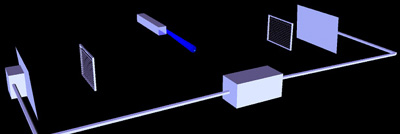Figure 42.8A: Apparatus
Simplification of the apparatus of the Aspect experiment including lasers (blue beam), polarizers (plates with lines running along each optical axis), detectors (smooth plates) and coincidence counter (box connected to both detectors).

See video clip of Aspect Experiment sequence with transmitted photons in MP4 format.

According to the quantum explanation, the polarization of each photon of a pair is not determined until a measurement is made, and the act of measuring the polarization of one photon causes an instantaneous action at a distance with regard to the measurement of the polarization of the other member of a given pair. Observations of correlated photon polarization is interpreted as proof of a spooky action at a distance. Thus, information travels faster than the speed of light in violation of special relativity, or nonlocality and noncausality are aspects of physical reality. Since there exists no independent results that support such a nonsensical prediction which is disproved by innumerable experiments as well as everyday experience, it is not surprising that a physical explanation exists. The sequence of events based on physical laws is shown in Figures 42.8A-E.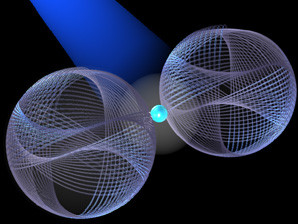Figure 42.8B: Photon Emission
The source current of the doubly-excited state atom gives rise to electromagnetic fields that become emitted photons in opposite directions wherein the radius of each photon is given by the ratio of the speed of light to the velocity change of the excited state electron upon de-excitation.

See video clip of Photon Emission in MP4 format.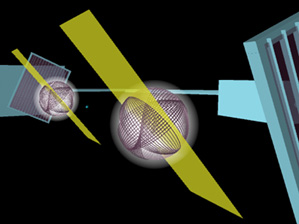Figure 42.8C: Polarization Correlation
The (yellow) plane of polarization of each photon pair is exactly correlated to conserve the angular momentum of the excited state.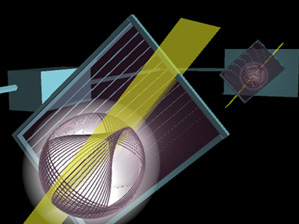Figure 42.8D: Transmitted Photons
The transmittance of each photon at each detector depends on the alignment or angle of the plane of polarization of the photons (random but matched) and the axis of each polarizer (rotated relative to each other by the experimenter). When the polarizers are parallel, the photons are both transmitted if each is sufficiently aligned with the polarizer.

See video clip of Aspect Experiment sequence with transmitted photons in MP4 format.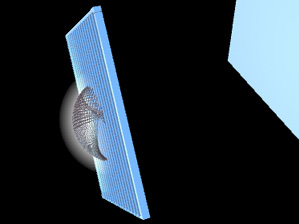Figure 42.8E: Blocked Photons
Or, both are blocked if the transmittance is low due to a condition of crossed polarization of each photon and polarizer. Intermediate cases depend on the relative angle of each photon and its polarizer as shown in Fig. 42.8C.

See video clip of Aspect Experiment sequence with blocked photons in MP4 format.

The expectation value of the coincidence rate at separated randomly oriented polarization analyzers for pairs of photons emitted from a doubly-excited state atom was derived from the equation of the photon. Rather than a point that obeys a probability-density wave, the photon is an extended particle with a finite radius given by the wavelength of the photon divided by 2*Pi. Consequently, the photon’s electric field vector has a projection onto the axis of each rotated polarizer’s axis. Angular momentum of the doubly excited-state atom is conserved by emitting photons of the same linear polarization in opposite directions. Thus, the photon polarization is exactly correlated based on physics.

Based on these physical attributes of the emitted photons, the normalized coincidence count rate, R(Phi)/R0, as a function of the relative polarizer orientation, Phi, is given by: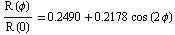which matches with the results of Aspect . The details are given in Chapter 42 of R. Mills.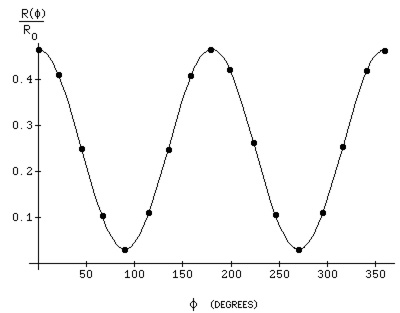Figure 42.9.
The normalized coincidence count rate as a function of the relative polarizer orientation (solid curve) with the results of Aspect  match. This result is based on the physical treatment of the photon as an extend particle that obeys Maxwell’s equations with conservation of angular momentum of the doubly-excited state calcium atom and the corresponding emission of two photons of the same linear polarization in opposite directions.

References
1. Aspect, A., Grangier, P., Gerard, R., Physical Review Letters, Vol. 47, No. 7, (1981), pp. 460-463.
2. Aspect, A., Grangier, P., Gerard, R., Physical Review Letters, Vol. 49, No. 2, (1982), pp. 91-94.
3. Aspect, A., Dalibard, J., , Gerard, R., Physical Review Letters, Vol. 49, No. 25, (1982), pp. 1804-1807.
4. Clauser, J., F., et al., Physical Review Letters, Vol. 23, No. 15, (1969), pp. 880-884.
5. Clauser, J., F., Horne, M., Physical Review D, Vol. 10, No. 2, (1974), pp. 526-535.
6. Horne, M., A., “Experimental Consequences of Local Hidden Variable Theories”, thesis, Boston University, (1969).
7. Mermin, N. D., Physics Today, April , (1995), pp. 38-47.
8. Mermin, N. D., Physics Today, June, (1994), pp. 9-11.
9. Mermin, N. D., Physics Today, June, (1990), pp. 9-11.
10. Einstein, A., Podolsky, B., Rosen, N., Phys. Rev., Vol. 47, (1935), p. 777.